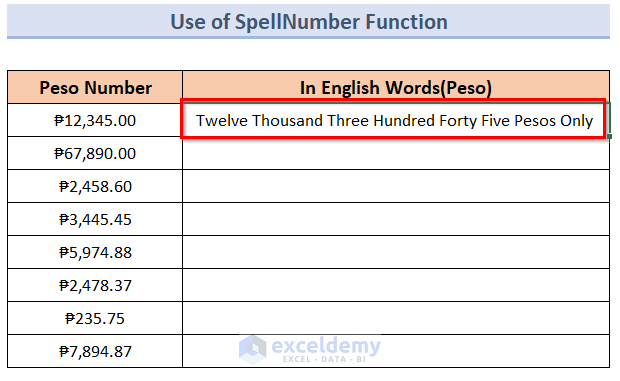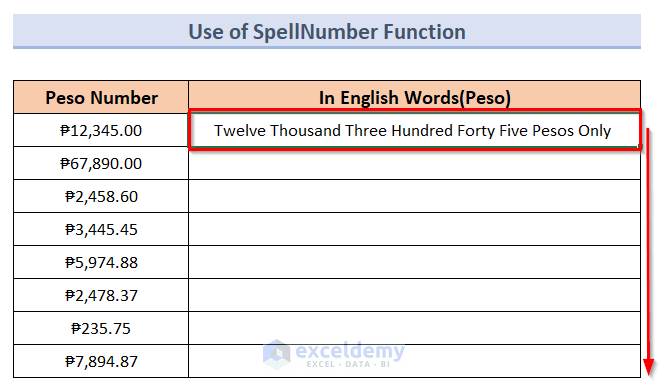# How to Convert Peso Number to Words in Excel (With Easy Steps)

Get FREE Advanced Excel Exercises with Solutions!

This tutorial will demonstrate how to convert peso number to words in Excel. Undoubtedly it is very important to convert the currency numbers into words while we are dealing with lots of cash records. In our scenario, we will try to convert peso numbers to words with the help of Excel. It can help to transact, display cash memos, and understand the debit credit easily in native or global persona.

## How to Convert Peso Number to Words in Excel: with Easy Steps

Our main goal is to follow some procedures to convert number to words in Excel in peso for a better understanding of any kind of cash records to any global person. Therefore, to achieve this goal, we have to follow the steps described below:

### Step 1: Arranging Dataset

To understand easily, we’ll use a sample dataset as an example in Excel. In this case, we have Peso Number in Column B and English Words(Peso) in Column C.### Step 2: Opening New Module for VBA Code

To use the VBA code in this situation we need to open the VBA window as described below:

• At this point, press Alt+F11 to open the Visual Basic For Applications option.
• Next, click on the Insert tab and select the Module option.### Step 3: Inserting VBA Code

• Afterward, insert the following VBA code into the window opened on the screen:
``````Option Explicit
Function SpellNumber(ByVal Number)
Dim Pesos, Cents, T
Dim Dec, Cnt
ReDim Position(9) As String
Position(2) = " Thousand "
Position(3) = " Million "
Position(4) = " Billion "
Position(5) = " Trillion "
Number = Trim(Str(Number))
Dec = InStr(Number, ".")
If Dec > 0 Then
Cents = GetTens2(Left(Mid(Number, Dec + 1) & _
"00", 2))
Number = Trim(Left(Number, Dec - 1))
End If
Cnt = 1
Do While Number <> ""
T = GetHundreds(Right(Number, 3))
If T <> "" Then Pesos = T & Position(Cnt) & Pesos
If Len(Number) > 3 Then
Number = Left(Number, Len(Number) - 3)
Else
Number = ""
End If
Cnt = Cnt + 1
Loop
Select Case Pesos
Case ""
Pesos = "No Pesos"
Case "One"
Pesos = "One Peso"
Case Else
Pesos = Pesos & ""
End Select
Select Case Cents
Case ""
Cents = " Pesos Only "
Case "One"
Cents = " and One Cent"
Case Else
Cents = " & " & Cents & "/100 Pesos Only"
End Select
SpellNumber = Pesos & Cents
End Function
Function GetHundreds(ByVal Number)
Dim R As String
If Val(Number) = 0 Then Exit Function
Number = Right("000" & Number, 3)
If Mid(Number, 1, 1) <> "0" Then
R = GetDigit(Mid(Number, 1, 1)) & " Hundred "
End If
If Mid(Number, 2, 1) <> "0" Then
R = R & GetTens(Mid(Number, 2))
Else
R = R & GetDigit(Mid(Number, 3))
End If
GetHundreds = R
End Function
Function GetTens(TensText)
Dim R As String
R = ""
If Val(Left(TensText, 1)) = 1 Then
Select Case Val(TensText)
Case 10: R = "Ten"
Case 11: R = "Eleven"
Case 12: R = "Twelve"
Case 13: R = "Thirteen"
Case 14: R = "Fourteen"
Case 15: R = "Fifteen"
Case 16: R = "Sixteen"
Case 17: R = "Seventeen"
Case 18: R = "Eighteen"
Case 19: R = "Nineteen"
Case Else
End Select
Else
Select Case Val(Left(TensText, 1))
Case 2: R = "Twenty "
Case 3: R = "Thirty "
Case 4: R = "Forty "
Case 5: R = "Fifty "
Case 6: R = "Sixty "
Case 7: R = "Seventy "
Case 8: R = "Eighty "
Case 9: R = "Ninety "
Case Else
End Select
R = R & GetDigit _
(Right(TensText, 1))
End If
GetTens = R
End Function
Function GetDigit(Digit)
Select Case Val(Digit)
Case 1: GetDigit = "One"
Case 2: GetDigit = "Two"
Case 3: GetDigit = "Three"
Case 4: GetDigit = "Four"
Case 5: GetDigit = "Five"
Case 6: GetDigit = "Six"
Case 7: GetDigit = "Seven"
Case 8: GetDigit = "Eight"
Case 9: GetDigit = "Nine"
Case Else: GetDigit = ""
End Select
End Function
Function GetTens2(TensText)
Dim R As String
R = ""
If Val(Left(TensText, 1)) = 1 Then
Select Case Val(TensText)
Case 10: R = "10"
Case 11: R = "11"
Case 12: R = "12"
Case 13: R = "13"
Case 14: R = "14"
Case 15: R = "15"
Case 16: R = "16"
Case 17: R = "17"
Case 18: R = "18"
Case 19: R = "19"
Case Else
End Select
Else
Select Case Val(Left(TensText, 1))
Case 2: R = "2"
Case 3: R = "3"
Case 4: R = "4"
Case 5: R = "5"
Case 6: R = "6"
Case 7: R = "7"
Case 8: R = "8"
Case 9: R = "9"
Case Else
End Select
R = R & GetDigit2 _
(Right(TensText, 1))
End If
GetTens2 = R
End Function
Function GetDigit2(Digit)
Select Case Val(Digit)
Case 0: GetDigit2 = "0"
Case 1: GetDigit2 = "1"
Case 2: GetDigit2 = "2"
Case 3: GetDigit2 = "3"
Case 4: GetDigit2 = "4"
Case 5: GetDigit2 = "5"
Case 6: GetDigit2 = "6"
Case 7: GetDigit2 = "7"
Case 8: GetDigit2 = "8"
Case 9: GetDigit2 = "9"
Case Else: GetDigit2 = ""
End Select
End Function``````### Step 4: Saving VBA Code

In this case, press Save As and select Excel Macro-Enabled Workbook(.xlsm) option to use the VBA code correctly.Read More: How to Use Spell Number in Excel

### Step 5: Using SpellNumber Function

The SpellNumber function is the executive function of the VBA code we prepared in the previous step. We can learn to use it in the following ways:

• After saving the VBA code press Alt+Q to return to the dataset.
• In the cell C5, type the following formula:
=SpellNumber(B5)Read More: How to Convert Number to Words in Excel Without VBA

### Step 6: Presenting Desired Result

• Afterward, press the Enter button to get the result like the below image.

##• Then select the cell, and drag it downwards.

##• Finally, you will get a result similar to the following image.Read More: How to Convert Number to Words in Excel in Rupees

## Convert Number to Words in Excel with Combination of Various Formulas

If you are not much comfortable with VBA code then you can use a combination of various formulas to convert number to words in Excel. The steps will be discussed below:

Steps:

• Firstly, arrange the dataset for the method.• Secondly, insert the following formula in cell C4, and press the enter button.

`=CHOOSE(LEFT(TEXT(B5,"000000000.00"))+1,,"One","Two","Three","Four","Five","Six","Seven","Eight","Nine")&IF(--LEFT(TEXT(B5,"000000000.00"))=0,,IF(AND(--MID(TEXT(B5,"000000000.00"),2,1)=0,--MID(TEXT(B5,"000000000.00"),3,1)=0)," Hundred"," Hundred and "))&CHOOSE(MID(TEXT(B5,"000000000.00"),2,1)+1,,,"Twenty ","Thirty ","Forty ","Fifty ","Sixty ","Seventy ","Eighty ","Ninety ")&IF(--MID(TEXT(B5,"000000000.00"),2,1)<>1,CHOOSE(MID(TEXT(B5,"000000000.00"),3,1)+1,,"One","Two","Three","Four","Five","Six","Seven","Eight","Nine"),CHOOSE(MID(TEXT(B8,"000000000.00"),3,1)+1,"Ten","Eleven","Twelve","Thirteen","Fourteen","Fifteen","Sixteen","Seventeen","Eighteen","Nineteen"))&IF((--LEFT(TEXT(B5,"000000000.00"))+MID(TEXT(B5,"000000000.00"),2,1)+MID(TEXT(B5,"000000000.00"),3,1))=0,,IF(AND((--MID(TEXT(B5,"000000000.00"),4,1)+MID(TEXT(B5,"000000000.00"),5,1)+MID(TEXT(B5,"000000000.00"),6,1)+MID(TEXT(B5,"000000000.00"),7,1))=0,(--MID(TEXT(B5,"000000000.00"),8,1)+RIGHT(TEXT(B5,"000000000.00")))>0)," Million and "," Million"))&CHOOSE(MID(TEXT(B5,"000000000.00"),4,1)+1,,"One","Two","Three","Four","Five","Six","Seven","Eight","Nine")&IF(--MID(TEXT(B5,"000000000.00"),4,1)=0,,IF(AND(--MID(TEXT(B5,"000000000.00"),5,1)=0,--MID(TEXT(B5,"000000000.00"),6,1)=0)," Hundred"," Hundred and"))&CHOOSE(MID(TEXT(B5,"000000000.00"),5,1)+1,,," Twenty"," Thirty"," Forty"," Fifty"," Sixty"," Seventy"," Eighty"," Ninety")&IF(--MID(TEXT(B5,"000000000.00"),5,1)<>1,CHOOSE(MID(TEXT(B5,"000000000.00"),6,1)+1,," One","Two","Three","Four","Five","Six","Seven","Eight","Nine"),CHOOSE(MID(TEXT(B5,"000000000.00"),6,1)+1," Ten"," Eleven"," Twelve"," Thirteen"," Fourteen"," Fifteen"," Sixteen"," Seventeen"," Eighteen"," Nineteen"))&IF((--MID(TEXT(B5,"000000000.00"),4,1)+MID(TEXT(B5,"000000000.00"),5,1)+MID(TEXT(B5,"000000000.00"),6,1))=0,,IF(OR((--MID(TEXT(B5,"000000000.00"),7,1)+MID(TEXT(B5,"000000000.00"),8,1)+MID(TEXT(B5,"000000000.00"),9,1))=0,--MID(TEXT(B5,"000000000.00"),7,1)<>0)," Thousand "," Thousand and "))&CHOOSE(MID(TEXT(B5,"000000000.00"),7,1)+1,,"One","Two","Three","Four","Five","Six","Seven","Eight","Nine")&IF(--MID(TEXT(B5,"000000000.00"),7,1)=0,,IF(AND(--MID(TEXT(B5,"000000000.00"),8,1)=0,--MID(TEXT(B5,"000000000.00"),9,1)=0)," Hundred "," Hundred and "))&CHOOSE(MID(TEXT(B5,"000000000.00"),8,1)+1,,,"Twenty ","Thirty ","Forty ","Fifty ","Sixty ","Seventy ","Eighty ","Ninety ")&IF(--MID(TEXT(B5,"000000000.00"),8,1)<>1,CHOOSE(MID(TEXT(B5,"000000000.00"),9,1)+1,,"One","Two","Three","Four","Five","Six","Seven","Eight","Nine"),CHOOSE(MID(TEXT(B5,"000000000.00"),9,1)+1,"Ten","Eleven","Twelve","Thirteen","Fourteen","Fifteen","Sixteen","Seventeen","Eighteen","Nineteen"))&" & "&RIGHT(TEXT(B5,"000000000.00"),2)&"/100"`• Thirdly, you will see the result for this certain cell.
• Then like in the below image drag the mouse downward.• Lastly, you will get the desired result as below.🔎 How Does the Formula Work?

This formula is a combination of various formulas. That is why it is important to describe every part of it for better understanding. This formula has four important elements such as:

The CHOOSE function is normally very useful in cases where high numbers of IF functions are involved in just one dataset. The main goal of using the CHOOSE function is to choose a certain value from a given number from a large number of values.

The structure of the function is:

=CHOOSE(index_num, value1, [value2], …)

The purpose of using the LEFT function is to mark and drag out a certain number of data, and numbers of data values or key phrases or characters from a certain position to the left side of the text.  The structure of the LEFT function is presented below:

=LEFT(text, [num_chars])

The main goal of applying the MID function is to extend a certain number of data values or numbers or characters from a certain side to the middle portion of the text. The MID function formula has the structure as below

=MID(text, start_num, num_chars)

In this structure,

• The text means the input cell.
• The start_num declares the position of a certain text dragging out in a certain direction.
• The num_chars represent the total number of data or key phrases or characters to drag them out.

The TEXT function presents the detailed formatting of one cell with a proper description. It has a structure like the below function.

=TEXT(value, format_text)

• CHOOSE(LEFT(TEXT(B5,”000000000.00″))+1,,”One”,”Two”,”Three”,”Four”,”Five”,”Six”,”Seven”,”Eight”,”Nine”)&IF(–LEFT(TEXT(B5,”000000000.00″))=0,,IF(AND(–MID(TEXT(B5,”000000000.00″),2,1)=0,–MID(TEXT(B5,”000000000.00″),3,1)=0),” Hundred”,” Hundred and “)): At the very beginning, we can see this portion of the formula. In this portion, we have to use the TEXT function to turn the number format into “00”.Then we move to the extended portion of the formula to have a more clear understanding of the result of this formula.
• CHOOSE(LEFT(TEXT(B5,”000000000.00″))+1,,”One”,”Two”,”Three”,”Four”,”Five”,”Six”,”Seven”,”Eight”,”Nine”)&IF(–LEFT(TEXT(B5,”000000000.00″))=0,,IF(AND(–MID(TEXT(B5,”000000000.00″),2,1)=0,–MID(TEXT(B5,”000000000.00″),3,1)=0),” Hundred”,” Hundred and “)): After this point, the above formula can give three types of results.
1. If the value is zero, then have crossed the million range and because of that nothing will come onto the screen.
2. But, If the afterward two digits are zero consistently, then “Hundred” writing will come onto the screen.
3. Otherwise, If the afterward two digits are not zero, then “Hundred and” writing will come onto the screen.

Read More: How to Convert Number to Words in Excel

## Things to Remember

• In this whole process, we have used the Excel Macro-Enabled Workbook(.xlsm) To use it on another device it is necessary to enable it every time. Otherwise, it won’t work.
• It is very important to save the VBA code. Otherwise, it won’t run.
• When using the SpellNumber Function, it is very important to focus on the cell you are using. Otherwise, it will create a lot of confusion.

## Conclusion

Henceforth, follow the above-described methods. Thus, you can learn how to convert peso number to words in Excel. Let us know if you have more ways to do the task.

Don’t forget to drop comments, suggestions, or queries if you have any in the comment section below.

## Related ArticlesHi there! I am Zehad Rian Jim. I graduated with a bachelor's degree in engineering from BUET. Currently, I am working as a technical content writer at ExcelDemy. You will find all my articles on Microsoft Excel on this site. Outside of the workplace, my hobbies and interests include watching movies, tv series, and meeting new people. I also enjoy sports. My favorite sports are Cricket (to watch and play) and Badminton (play).

1. ReplyNothing left to us to give you thanks. You are Greate in all regards of Excel.

• ReplyThanks Akash!
-Mahdy
ExcelDemy team

2. ReplyAmazing!!! Thanjk you!

• ReplyShamima Sultana Aug 10, 2023 at 3:30 PM

Dear Karen,

You are most welcome.

Regards
ExcelDemyAdvanced Excel Exercises with Solutions PDF